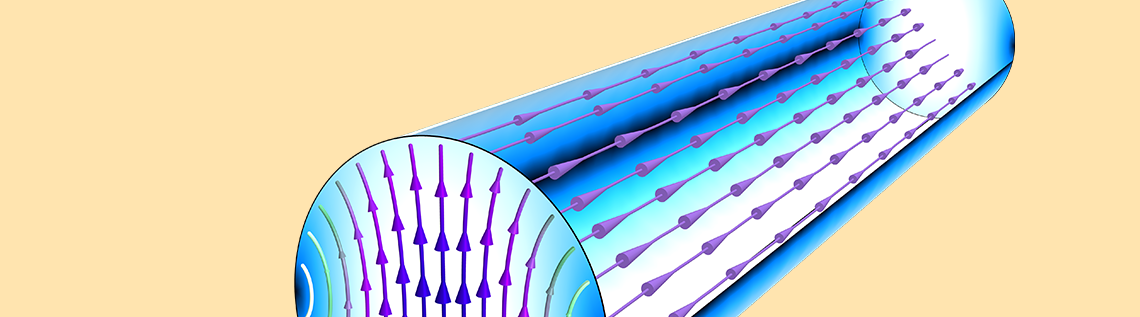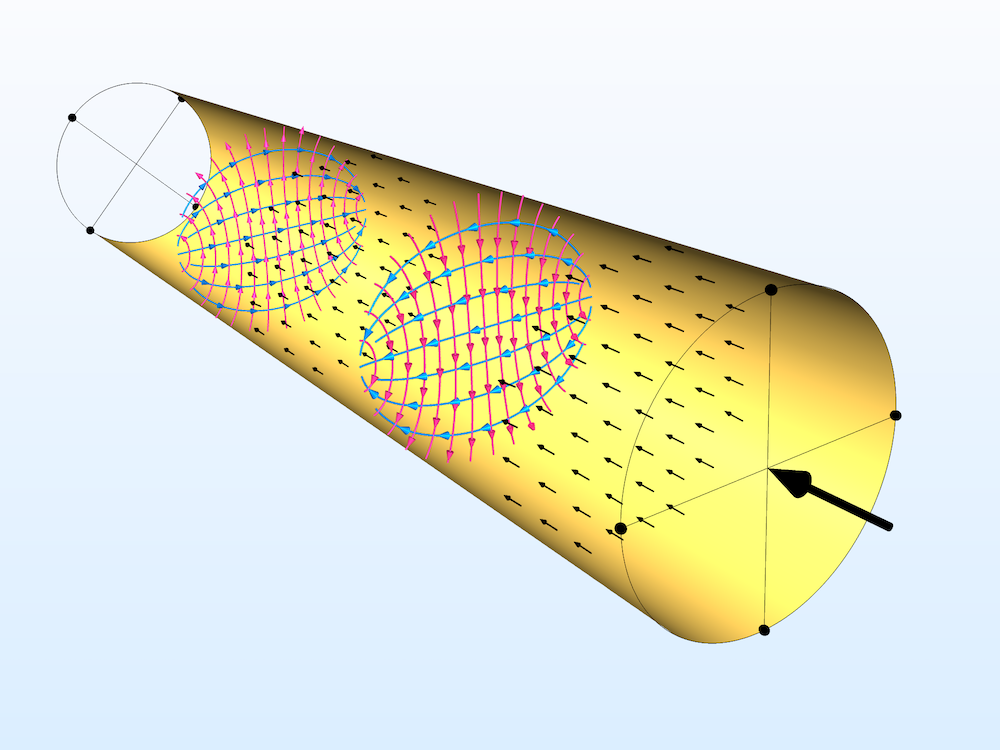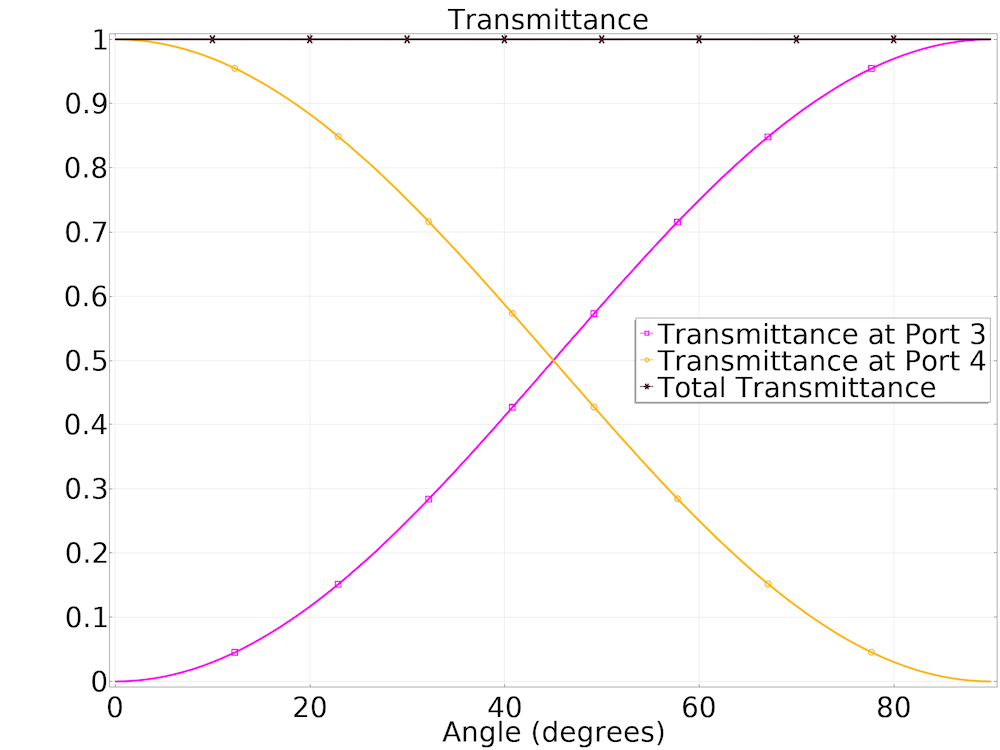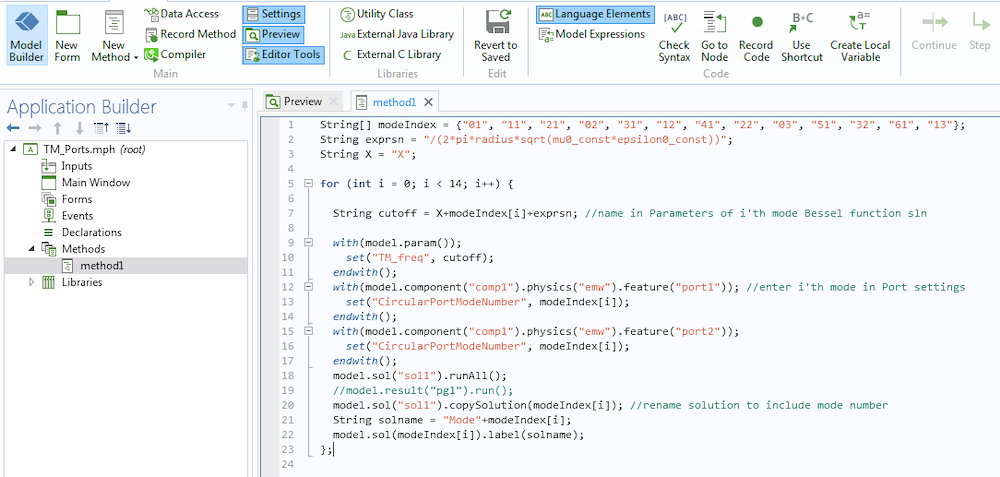# 如何在 RF 模块中使用圆形端口

2019年 5月 10日

RF 模块中的 端口 边界条件是 COMSOL Multiphysics® 软件的附加功能，可用于发射和吸收电磁能。在本篇博客中，我们将介绍如何设置一个圆形波导端口，并检查定义端口模场的解析解。另外，我们还从端口方向分析了一个用于功率传输的圆形端口偏振，并将模型扩展到高阶模式。

### 用于描述简并模式的圆形端口参考轴

E_{\rho}=\frac{-j\omega\mu m}{k_{c}^{2}\rho}(A \ cos \ m\phi-B \ sin \ m\phi) \ J_{m}(k_{c}\rho) \ e^{-j\beta z}E_{\phi}=\frac{j\omega\mu}{k_{c}}(A \ sin \ m\phi+B \ cos \ m\phi) \ J’_{m}(k_{c}\rho) \ e^{-j\beta z}

H_{\rho}=\frac{-j\beta}{k_{c}}(A \ sin \ m\phi+B \ cos \ m\phi) \ J’_{m}(k_{c}\rho) \ e^{-j\beta z}H_{\phi}=\frac{-j\beta m}{k_{c}^{2}\rho}(A \ cos \ m\phi-B \ sin \ m\phi) \ J_{m}(k_{c}\rho) \ e^{-j\beta z}TE11 模通过圆形波导传播。等值线图的动画显示了 E 场的 z 分量，该分量是使用完整的动态数据扩展序列类型创建的。箭头图描述了端口边界上的电模场。

### 模拟圆形波导偏振### 一个重要因素：截止频率

f_c=\frac{\chi'{mn}}{2\pi a \sqrt{\mu\epsilon}}，用于 TE 模式
f_c=\frac{\chi{mn}}{2\pi a \sqrt{\mu\epsilon}}，用于 TM 模式

χ’mn 的值通过贝塞尔函数 Jm(x) 的导数的零点给出，该值是确定 TE 模式的截止所必需的。χmn 的值由贝塞尔函数的零点给出，该值为确定TM模式的截止所必需的。一般情况下，贝塞尔函数的零点及其一阶导数都是已知的，下面列出了其中的一些值。

n = 1 2.4049 3.8318 5.1357 6.3802 7.5884 8.7715
n = 2 5.5201 7.0156 8.4173 9.7610 11.0647 12.3386
n = 3 8.6537 10.1735 11.6199 13.0152 14.3726 15.7002

n = 1 3.8318 1.8412 3.0542 4.2012 5.3175 6.4155
n = 2 7.0156 5.3315 6.7062 8.0153 9.2824 10.5199
n = 3 10.1735 8.5363 9.9695 11.3459 12.6819 13.9872

#### 使用“方法”工具自动收集数据

1. 计算给定模式的截止频率
2. 端口 节点设置中输入模式编号
3. 以略高于截止的频率值运行模型，并将解存储在端口边界### 后续操作

1. David M. Pozar, Microwave Engineering, John Wiley & Sons, 1998.
2. Constantine A. Balanis, Advanced Engineering Electromagnetics, John Wiley & Sons, 1999.